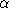# 3D Alpha ShapesReference Manual

Tran Kai Frank Da and Mariette Yvinec

Alpha shapes definition is based on an underlying triangulation that may be a Delaunay triangulation in case of basic alpha shapes or a regular triangulation (cf. 27.3) in case of weighted alpha shapes.

Let us consider the basic case with a Delaunay triangulation. We first define the alpha complex of the set of points S. The alpha complex is a subcomplex of the Delaunay triangulation. For a given value of, the alpha complex includes all the simplices in the Delaunay triangulation which have an empty circumsphere with squared radius equal or smaller than. Here ``empty'' means that the open sphere do not include any points of S. The alpha shape is then simply the domain covered by the simplices of the alpha complex (see [EM94]).

In general, an alpha complex is a non-connected and non-pure complex. This means in particular that the alpha complex may have singular faces. For 0kd-1, a k-simplex of the alpha complex is said to be singular if it is not a facet of a (k+1)-simplex of the complex CGAL provides two versions of the alpha shapes. In the general mode, the alpha shapes correspond strictly to the above definition. The regularized mode provides a regularized version of the alpha shapes corresponding to the domain covered by a regularized version of the alpha complex where singular faces are removed.

The alpha shapes of a set of points S form a discrete family, even though they are defined for all real numbers. The entire family of alpha shapes can be represented through the underlying triangulation of S. In this representation each k-simplex of the underlying triangulation is associated with an interval that specifies for which values ofthe k-simplex belongs to the alpha complex. Relying on this fact, the family of alpha shapes can be computed efficiently and relatively easily. Furthermore, we can select the optimal value ofto get an alpha shape including all data points and having less than a given number of connected components.

The definition is analog in the case of weighted alpha shapes. The input set is now a set of weighted points (which can be regarded as spheres) and the underlying triangulation is the regular triangulation of this set. Two spheres, or two weighted points , with centers C1, C2 and radii r1, r2 are said to be orthogonal iff  C1C2 2 = r12 + r22 and suborthogonal iff  C1C2 2 < r12 + r22. For a given value ofthe weighted alpha complex is formed with the simplices of the regular triangulation triangulation such that there is a sphere orthogonal to the weighted points associated with the vertices of the simplex and suborthogonal to all the other input weighted points. Once again the alpha shape is then defined as the domain covered by a the alpha complex and arise in two versions general or regularized.

## 30.5   Classified Reference Pages

### Classes

CGAL::Alpha_status<NT>
CGAL::Alpha_shape_3<Dt>
CGAL::Weighted_alpha_shape_euclidean_traits_3<K>
CGAL::Alpha_shape_vertex_base_3<Traits,Vb>
CGAL::Alpha_shape_cell_base_3<Traits,Fb>